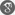##### Cite as:
K.R. Prasad, B.M.B. Krushna, L.T. Wesen, Existence of Positive Solutions for a Coupled System of (p, q)-Laplacian Fractional Higher Order Boundary Value Problems, Int. J. Anal. Appl., 9 (1) (2015), 54-67.

#### Abstract

In this paper, we establish the existence of at least three positive solutions for a system of (p,q)-Laplacian fractional order two-point boundary value problems by applying five functionals fixed point theorem under suitable conditions on a cone in a Banach space.

#### References

1. R. P. Agarwal, D. O’Regan and P. J. Y. Wong, Positive Solutions of Differential, Difference and Integral Equations, Kluwer Academic Publishers, Dordrecht, The Netherlands, 1999.2. D. R. Anderson and J. M. Davis, Multiple positive solutions and eigenvalues for third order right focal boundary value problems, J. Math. Anal. Appl., 267(2002), 135–157.3. R. I. Avery, A generalization of the Leggett-Williams fixed point theorem, Math. Sci. Res. Hot-line, 3(1999), 9–14.4. R. I. Avery and J. Henderson, Existence of three positive pseudo-symmetric solutions for a onedimensional p-Laplacian, J. Math. Anal. Appl., 277(2003), 395–404.5. C. Bai, Existence of positive solutions for boundary value problems of fractional functional differential equations, Elec. J. Qual. Theory Diff. Equ., 30(2010), 1–14.6. Z. Bai and H. L¨u, Positive solutions for boundary value problem of nonlinear fractional differential equation, J. Math. Anal. Appl., 311(2005), 495-505.7. G. Chai, Positive solutions for boundary value problem of fractional differential equation with pLaplacian operator, Bound. Value Probl., 2012(2012), 1–18.8. T. Chen and W. Liu, An anti-periodic boundary value problem for the fractional differential equation with a p-Laplacian operator, Appl. Math. Lett., 25(2012), 1671–1675.9. R. Dehghani and K. Ghanbari, Triple positive solutions for boundary value problem of a nonlinear fractional differential equation, Bulletin of the Iranian Mathematical Society, 33(2007), 1–14.10. L. Diening, P. Lindqvist and B. Kawohl, Mini-Workshop: The p-Laplacian Operator and Applications, Oberwolfach Reports, 10(2013) 433–482.11. L. H. Erbe and H. Wang, On the existence of positive solutions of ordinary differential equations, Proc. Amer. Math. Soc., 120(1994), 743–748.12. C. Goodrich, Existence of a positive solution to systems of differential equations of fractional order, Comput. Math. Appl., 62(2011), 1251–1268.13. D. Guo, V. Lakshmikantham, Nonlinear Problems in Abstract Cones, Acadamic Press, San Diego, 1988.14. J. Henderson and S. K. Ntouyas, Positive solutions for systems of nonlinear boundary value problems, Nonlinear Stud., 15(2008), 51-60.15. A. A. Kilbas, H. M. Srivasthava and J. J. Trujillo, Theory and Applications of Fractional Differential Equations, North-Holland Mathematics Studies, vol. 204, Elsevier Science, Amsterdam, 2006.16. L. Kong and J. Wang, Multiple positive solutions for the one-dimensional p-Laplacian, Nonlinear Anal., 42(2000), 1327–1333.17. I. Podulbny, Fractional Diffrential Equations, Academic Press, San Diego, 1999.18. K. R. Prasad and B. M. B. Krushna, Multiple positive solutions for a coupled system of p-Laplacian fractional order two-point boundary value problems, Int. J. Differ. Equ., 2014(2014), Article ID 485647, 1–10.19. K. R. Prasad and B. M. B. Krushna, Multiple positive solutions for the system of (n, p)-type fractional order boundary value problems, Bull. Int. Math. Virtual Inst., 5(2015), 1–12.20. K. R. Prasad and B. M. B. Krushna, Solvability of p-Laplacian fractional higher order two-point boundary value problems, Commun. Appl. Anal., 19(2015), 659–678.21. X. Su, Boundary value problem for a coupled system of nonlinear fractional differential equations, Appl. Math. Lett., 22(2009), 64–69.22. C. Yang, J. Yan, Positive solutions for third order Sturm–Liouville boundary value problems with p-Laplacian, Comput. Math. Appl., 59(2010), 2059–2066.